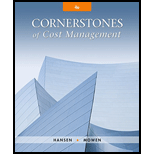# Head-Gear Company produces helmets for bicycle racing. Currently, Head-Gear charges a price of $230 per helmet. Variable costs are$80.50 per helmet, and fixed costs are $1,255,800. The tax rate is 25 percent. Last year, 14,000 helmets were sold. Required: 1. What is Head-Gear’s net income for last year? 2. What is Head-Gear’s break-even revenue? (Round to the nearest dollar.) 3. Suppose Head-Gear wants to earn before-tax operating income of$900,000. How many units must be sold? (Round to the nearest unit.) 4. Suppose Head-Gear wants to earn after-tax net income of $650,000. How many units must be sold? (Round to the nearest unit.) 5. Suppose the income tax rate rises to 35 percent. How many units must be sold for Head-Gear to earn after-tax income of$650,000? (Round to the nearest unit.)### Cornerstones of Cost Management (C...

4th Edition
Don R. Hansen + 1 other
Publisher: Cengage Learning
ISBN: 9781305970663

#### Solutions

Chapter
Section### Cornerstones of Cost Management (C...

4th Edition
Don R. Hansen + 1 other
Publisher: Cengage Learning
ISBN: 9781305970663
Chapter 16, Problem 16E
Textbook Problem
46 views

## Head-Gear Company produces helmets for bicycle racing. Currently, Head-Gear charges a price of $230 per helmet. Variable costs are$80.50 per helmet, and fixed costs are $1,255,800. The tax rate is 25 percent. Last year, 14,000 helmets were sold.Required: 1. What is Head-Gear’s net income for last year? 2. What is Head-Gear’s break-even revenue? (Round to the nearest dollar.) 3. Suppose Head-Gear wants to earn before-tax operating income of$900,000. How many units must be sold? (Round to the nearest unit.) 4. Suppose Head-Gear wants to earn after-tax net income of $650,000. How many units must be sold? (Round to the nearest unit.) 5. Suppose the income tax rate rises to 35 percent. How many units must be sold for Head-Gear to earn after-tax income of$650,000? (Round to the nearest unit.)

1.

To determine

Compute the net income for the previous year.

### Explanation of Solution

Net income: The bottom line of income statement which is the result of excess of earnings from operations (revenues) over the costs incurred for earning revenues (expenses) is referred to as net income.

Compute the net income for the previous year:

 For the previous year Particulars Amount ($) Sales (1)$3,220,000 Less: Variable cost (2) $1,127,000 Contribution margin$2,093,000 Less: Fixed cost $1,255,800 Operating income$837,200 Less: Income taxes (3) $209,300 Net income$627,900

Table (1)

The net income for the previous year is $627,900. Working Note (1): Compute the value of sales: Sales=Unit Cost × Number of units sold 2. To determine Compute the break-even in sales revenue. 3. To determine Compute the number of units to be sold to generate the before-tax operating income of$900,000.

4.

To determine

Compute the number of units to be sold to generate the after-tax income of $650,000. 5. To determine Compute the number of units to be sold to generate the after-tax income of$650,000, if the tax rate increases.

### Still sussing out bartleby?

Check out a sample textbook solution.

See a sample solution

#### The Solution to Your Study Problems

Bartleby provides explanations to thousands of textbook problems written by our experts, many with advanced degrees!

Get Started

Find more solutions based on key concepts
Outline the historical evolution of business social responsibility.

Foundations of Business (MindTap Course List)

What, in your own words, does BR entail?

Pkg Acc Infor Systems MS VISIO CD

Why cant the Fed control the money supply perfectly?

Principles of Macroeconomics (MindTap Course List)

RISK ANALYSIS a. Given the following information, calculate the expected value for Firm C's EPS. Data for Firms...

Fundamentals of Financial Management, Concise Edition (with Thomson ONE - Business School Edition, 1 term (6 months) Printed Access Card) (MindTap Course List)

Discuss target market strategies

MKTG 12:STUDENT ED.-TEXT